# Mathematics - Signals And Systems - Discrete-Time Fourier Transform Properties

in StemSociallast month[Image1]

## Introduction

Hey it's a me again @drifter1!

Today we continue with my mathematics series about Signals and Systems in order to cover Discrete-Time Fourier Transform Properties.

So, without further ado, let's dive straight into it!

## Discrete-Time Fourier Transform Usefulness

The discrete-time Fourier transform has mostly the same properties as the continous-time Fourier transform. A major difference between the two is that the discrete-time Fourier transform always results in a periodic function of Ω. In addition to that, the discrete-time Fourier transform is also specified completely over a range of . In the case of real-valued sequences ranges like (0, π) are also sufficient, because of conjugate symmetry.

Let's recall the two major equations of the Discrete-Time Fourier Transform. The first one, known as the synthesis equation, is defined as follows: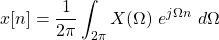Because this equations takes in X(Ω) and gives back the original signal x[n], its most commonly referenced as the Inverse Fourier Transform.

The analysis equation or Fourier Transform is defined as follows: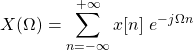As such, this equation takes in the original signal x[n] and gives the coefficients X(Ω).

Its also common to denote these two transformations as follows: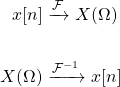Let's cover the various properties of the Discrete-Time Fourier Transform.

## Periodicity

Because the discrete-time Fourier transform results in a periodic function of Ω, periodicity becomes a very useful property. As mentioned before, the Fourier transform has a period of , which means that the Fourier transform at Ω is the same as the one at Ω + 2π, Ω + 2⋅2π, etc, or: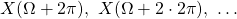Thus, for any integer m the following is true: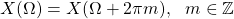## Linearity

Similar to continuous-time, the Fourier Transform of discrete-time also satisfies linearity. As such, the following is true, for any number of weighted signals: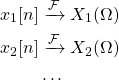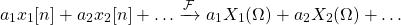## Time and Frequency Shifting

Using specific substitutions on the analysis and synthesis equations of the discrete-time Fourier transform, its possible to specify time and frequency shifting properties.

More specifically, using x[n - no] instead of x[n] in the analysis equation leads to the time-shifting property: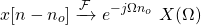Similarly, a substitution of X(Ω) with Χ(Ω - Ωo) leads to frequency-shifting, which is defined as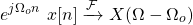## Conjugation and Conjugate Symmetry

As mentioned before, the discrete-time fourier transform also satisfies conjucation and conjugate symmetry. The result of the Fourier Transform, X(Ω), can be re-written as: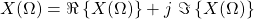When the signal x[n] is real, conjugate symmetry is satisfied, which means: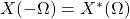because the real part is even and the imaginary part is odd: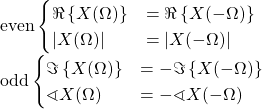## Differencing and Accumulation (basically Differentation and Integration in Discrete-Time)

In Discrete-Time there is no differentation and integration, but its possible to define similar things.

Differentiation can be thought of as the difference between a pair of Fourier transforms, basically x[n] - x[n - 1], which leads us to the differencing property: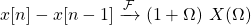Accumulation is the analog of Integration and defined as follows: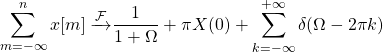I'm not quite sure about these two equations, as the notation was quite different in my two references (e = Ω in MIT/Openheim for the most part) and the MIT notes skip these two properties.

## Time Reversal and Expansion

Next up are the properties for time reversal and expansion.

Similar to continuous-time, the time-reversal of x[n], x[-n], leads to the inversion of the Fourier transform as well, or in maths: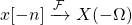In discrete-time time scaling is known as time expansion. This process is more difficult to replicate in discrete-time. Parallelizing a signal x[n] by a factor k leads to a signal x[n / k]. This signal is non-zero only when n is a multiple of k (or n / k is an integer). Oppenheim denotes this signal as x(k)[n].

Let's summarize the definition of the time-expanded signal: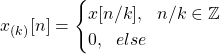The corresponding Fourier transform of such a signal is given by the equation: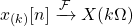## Differentiation in Frequency

In addition to the accumulation and differencing properties of time, its also possible to define the derivative in frequency. Differentiating both sides of the analysis equations by Ω, and multiplying by j leads to the following property: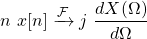Let's note that integration is not defined in frequency.

## Parseval's Relation

Parseval's relation can also be defined for discrete-time.

For any Fourier transform pair x[n] and X(Ω) the following is true: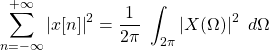The square of the Fourier transform X(Ω) is referred to as the energy density spectrum of signal x[n]. Integrating from to yields finite energy, which tells us that x[n] has finite energy.

## Convolution and Multiplication

Exactly like in continuous-time, the Fourier transform of the convolution of two discrete-time signals is equal to the multiplication of their individual Fourier transforms. Mathematically, in the case of a generic LTI system with input x[n] and impulse response h[n], its possible to define the output y[n] and its Fourier Transform Y[Ω] as follows: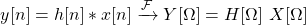Similar to continuous-time, multiplication is again known as modulation, with the result being the convolution of the individual Fourier transforms. The main difference is that the convolution is now periodic, and not aperiodic. Mathematically, the modulation of the signals x[n] and y[n] is defined as follows: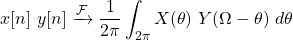## Duality in Discrete-Time

There is no duality between the discrete-time Fourier transform synthesis and analysis equations, but there is one amongst the Fourier series equations of discrete-time. A duality relation also exists between the discrete-time Fourier transform and continuous-time Fourier series.

### Duality between the Discrete-Time Fourier Series equations

In the case of continuous-time periodic signals there is an inherent asymmetry which yields a lack of duality between the time and frequency domains. On the other hand, aperiodic signals show a strong duality, with the Fourier transform of the Fourier transform returning the original signal, but time-reversed.

Let's now recall the discrete-time Fourier series equations: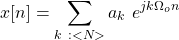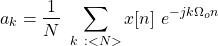In the case of the discrete-time domain, the situation is exactly opposite. The Fourier series represents a periodic sequence using a periodic sequence of Fourier series coefficients. In contrast to that, the Fourier transform represents an aperiodic sequence using a continuous periodic function, its Fourier transform. Thus, only the Fourier series equations show a strong duality, which means that every property of the discrete-time Fourier series has a dual.

For example, one pair is time-shifting and frequency-shifting: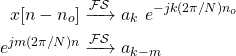### Duality between the Discrete-Time Fourier Transform and Continuous-Time Fourier Series

There also exists a strong duality between the continuous-time Fourier series and the discrete-time Fourier transform. Its easy to notice this relationship by comparing the analysis equation of the Fourier series and the synthesis equation of the Fourier transform, or vise versa: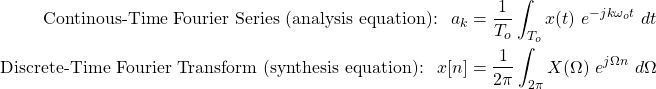In conclusion, there are three dualities in total:

• Between the continous-time Fourier transform analysis and synthesis equations
• Between the discrete-time Fourier series analysis and synthesis equations
• Between the continous-time Fourier series and discrete-time Fourier transform equations (synthesis to analysis)

## RESOURCES:

### Images

Block diagrams and other visualizations were made using draw.io

## Final words | Next up

And this is actually it for today's post!

I'm thinking of getting into examples/exercises of some sort for the topics that we covered!

See Ya!Keep on drifting!

Sort:Thanks for your contribution to the STEMsocial community. Feel free to join us on discord to get to know the rest of us!

Please consider supporting our funding proposal, approving our witness (@stem.witness) or delegating to the @stemsocial account (for some ROI).

Please consider using the STEMsocial app app and including @stemsocial as a beneficiary to get a stronger support.